Publicité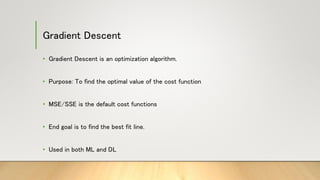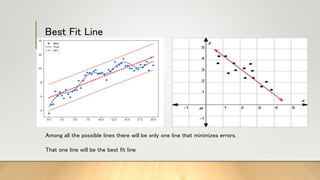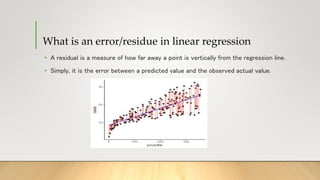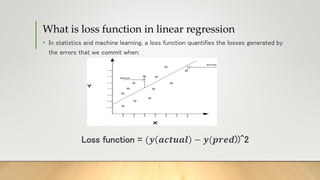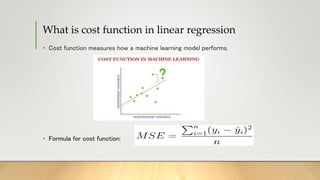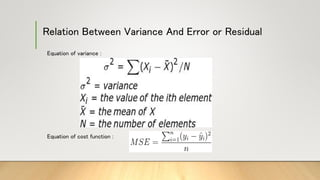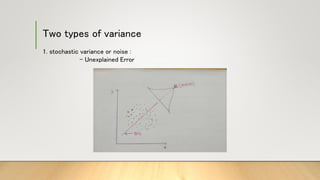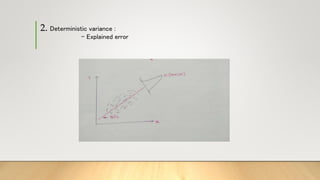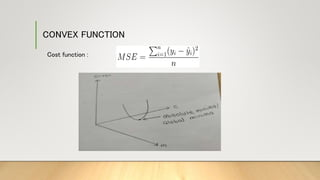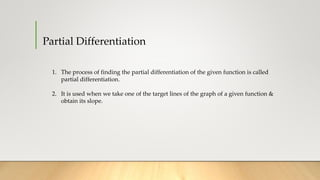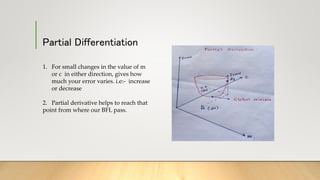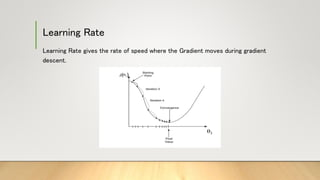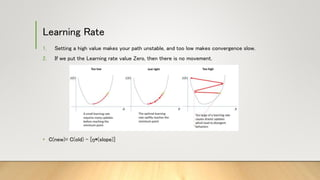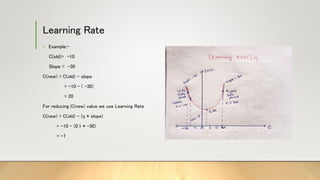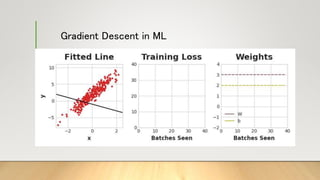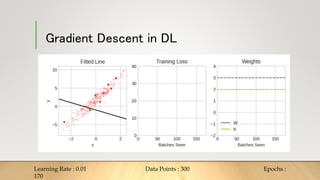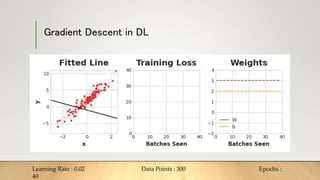1 sur 19
Publicité

1. Gradient Descent • Gradient Descent is an optimization algorithm. • Purpose: To find the optimal value of the cost function • MSE/SSE is the default cost functions • End goal is to find the best fit line. • Used in both ML and DL
2. Best Fit Line Among all the possible lines there will be only one line that minimizes errors. That one line will be the best fit line
3. What is an error/residue in linear regression • A residual is a measure of how far away a point is vertically from the regression line. • Simply, it is the error between a predicted value and the observed actual value.
4. What is loss function in linear regression • In statistics and machine learning, a loss function quantifies the losses generated by the errors that we commit when: Loss function = (𝒚 𝒂𝒄𝒕𝒖𝒂𝒍 − 𝒚(𝒑𝒓𝒆𝒅))^2
5. Types of loss function • Mean Absolute Error (MAE). • Mean Absolute Percentage Error (MAPE). • Mean Squared Error (MSE). • Root Mean Squared Error (RMSE). • Huber Loss. • Log-Cosh Loss.
6. What is cost function in linear regression • Cost function measures how a machine learning model performs. • Formula for cost function:
7. Relation Between Variance And Error or Residual Equation of variance : Equation of cost function :
8. Two types of variance 1. stochastic variance or noise : - Unexplained Error
9. 2. Deterministic variance : - Explained error
10. CONVEX FUNCTION Cost function :
11. Partial Differentiation 1. The process of finding the partial differentiation of the given function is called partial differentiation. 2. It is used when we take one of the target lines of the graph of a given function & obtain its slope.
12. Partial Differentiation 1. For small changes in the value of m or c in either direction, gives how much your error varies. i.e:- increase or decrease 2. Partial derivative helps to reach that point from where our BFL pass.
13. Learning Rate Learning Rate gives the rate of speed where the Gradient moves during gradient descent.
14. Learning Rate 1. Setting a high value makes your path unstable, and too low makes convergence slow. 2. If we put the Learning rate value Zero, then there is no movement. • C(new)= C(old) – [ŋ*(slope)]
15. Learning Rate • Example:- C(old)= -10 Slope = -30 C(new) = C(old) – slope = -10 - ( -30) = 20 For reducing C(new) value we use Learning Rate C(new) = C(old) - (ŋ * slope) = -10 - (0.1 * -30) = -7